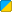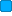# Entity.PhysicsInitBox

 Function Syntax Entity:PhysicsInitBox( Vector min, Vector max ) Description: In a scripted entity's initilization event, this function will give the entity the physics model of a box. The box's corners are min and max (see additional notes for more information). Returns: nil In Object: Entity Realm:BBCode Link: [b][url=http://wiki.garrysmod.com/?title=Entity.PhysicsInitBox]Entity.PhysicsInitBox [img]http://wiki.garrysmod.com/favicon.ico[/img][/url][/b]

## Examples

Description Create a physics entity with a 10x10x10 box physics model.```  function ENT:Initialize() local w=10 //Width local l=10 //Length local h=10 //Height   //Vectors local min=Vector(0-(w/2),0-(l/2),0-(h/2)) local max=Vector(w/2,l/2,h/2)   //Give it some kind of model self:SetModel("models/BarneyHelmet_faceplate.mdl")   //Set physics box self:PhysicsInitBox(min,max)   //Set bounding box - this will be used for triggers and //determining if rendering is necessary clientside) self:SetCollisionBounds(min,max) end  ``` N/A

• The physics box remains at a constant angle to the world, meaning it doesn't rotate with the model.
• Both of the vectors should be local vectors (meaning that 0,0,0 is the center of the model, not the center of the map)
• The vectors are measured in world units.
• The 'min' is the lowest X, Y, and Z values on the model. For a normal box, all three values should be the same as 'max', but negative.
• The 'max' is the highest X, Y, and Z values on the model. For a normal box, all three values should be the same as 'min', but positive.